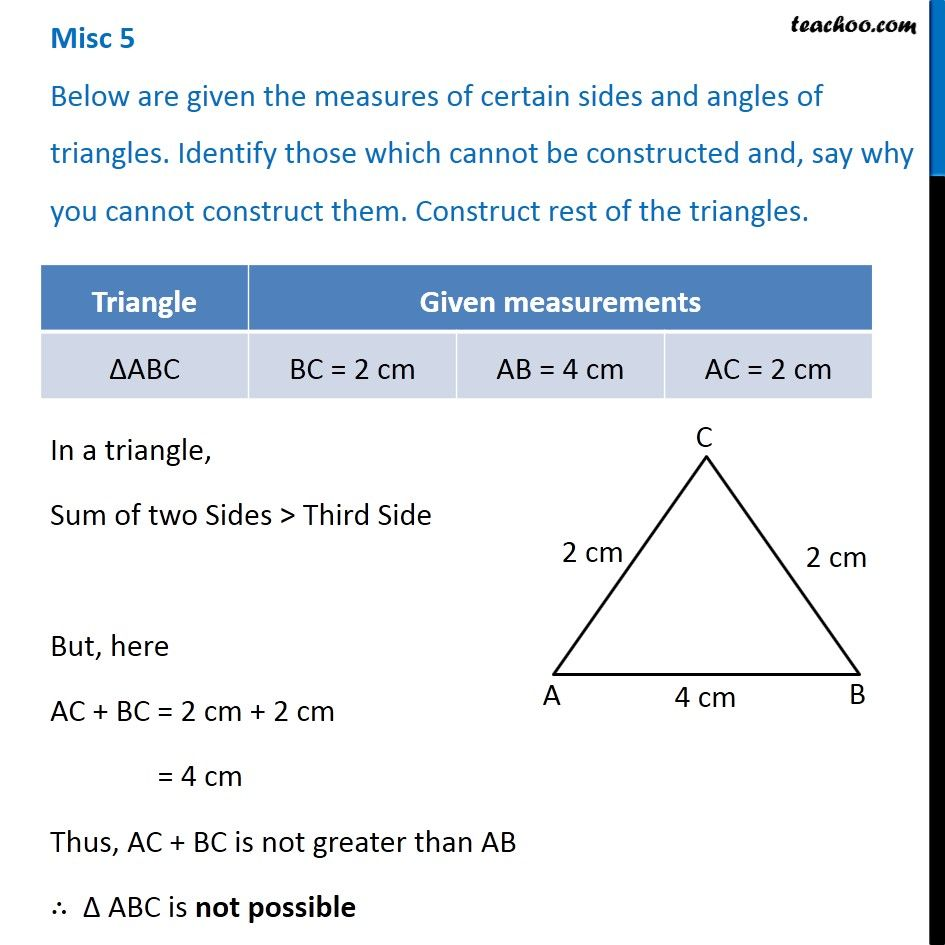1. Chapter 10 Class 7 Practical Geometry
2. Concept wise
3. Constructing a triangle when 3 sides known (SSS)

Transcript

Misc 5 Below are given the measures of certain sides and angles of triangles. Identify those which cannot be constructed and, say why you cannot construct them. Construct rest of the triangles. In a triangle, Sum of two Sides > Third Side But, here AC + BC = 2 cm + 2 cm = 4 cm Thus, AC + BC is not greater than AB ∴ Δ ABC is not possible

Constructing a triangle when 3 sides known (SSS)